# How can I estimate the minimum current measurement of a current probe using an oscilloscope?

September 27, 2021

Here is a quick method for estimating the lowest current measurement that is possible with an oscilloscope and current probe. This is especially useful when the datasheet for a probe may not show the minimum current that can be measured or it may be missing the noise floor value.

For this note, we will be using the SIGLENT CP4020 current probe and the SDS1104X-E oscilloscope as well as an SPD1168X power supply.

1. Let’s check the noise floor of the scope. The SDS1104X-E has a 500 uV/division minimum scale. The channel noise (with no input connection/CH1 open) is approximately 1 division (500 uV p-p):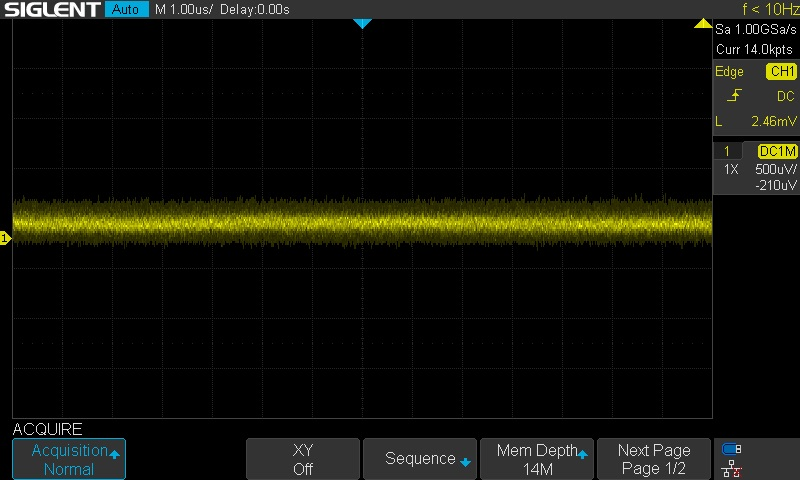2. Now, let’s connect the probe, turn it on, and switch the gain to the most sensitive setting. In this case, 50 mV/A: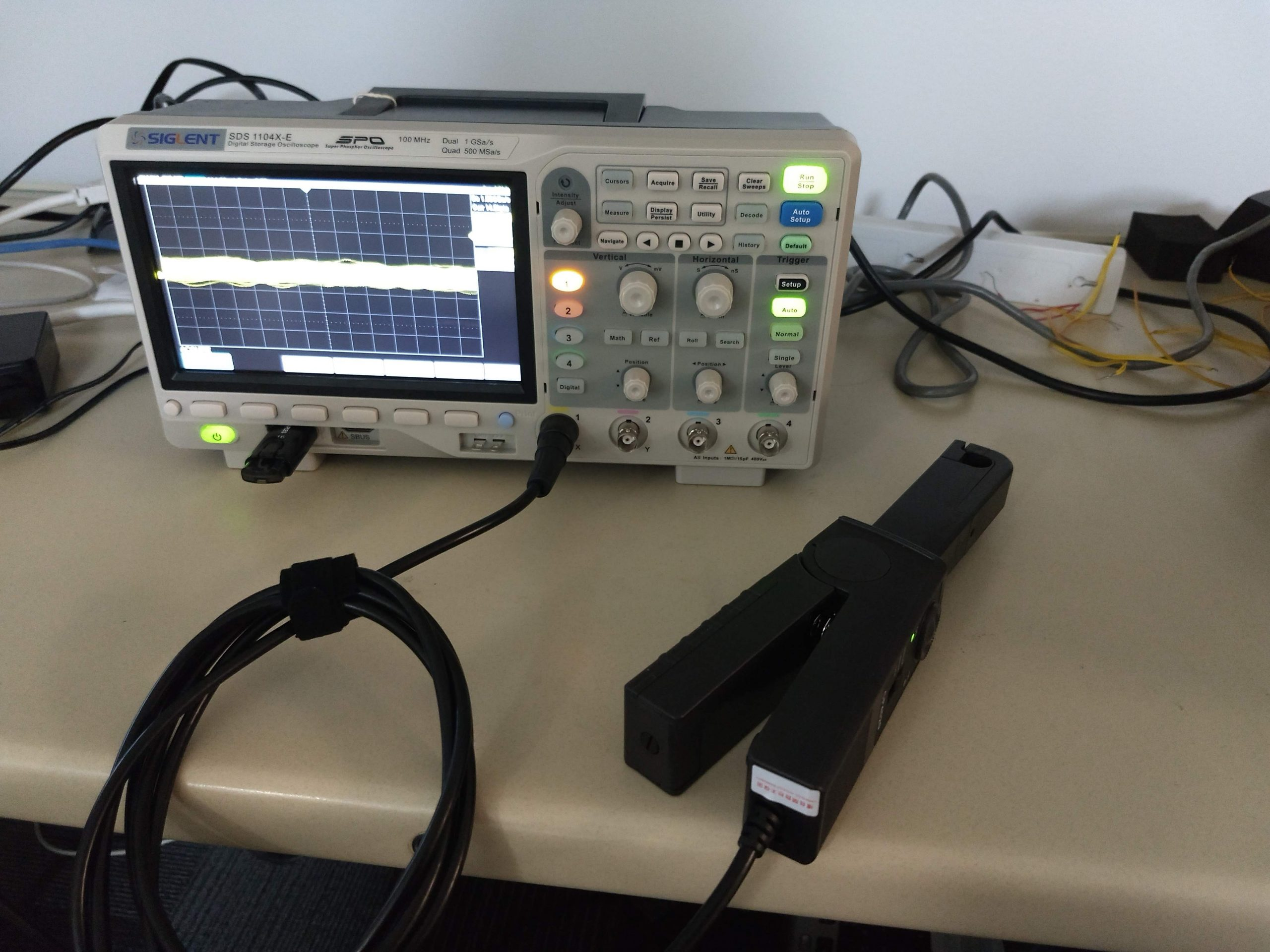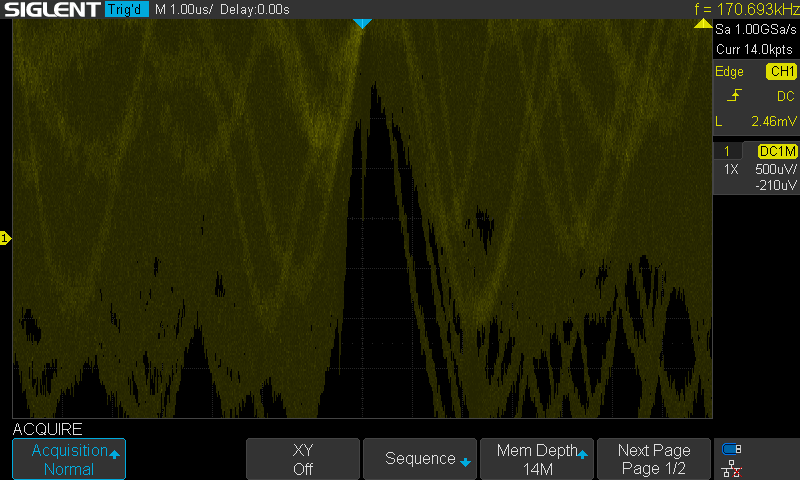The noise on the 500 uV/div vertical scale is dominating the screen.. so we can adjust the scale until we get some headroom… 5 mV/div is better..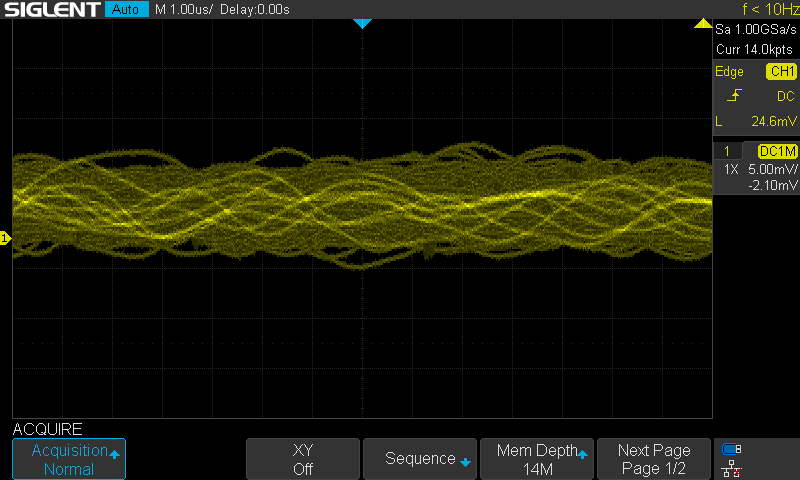10 mV/div even more so.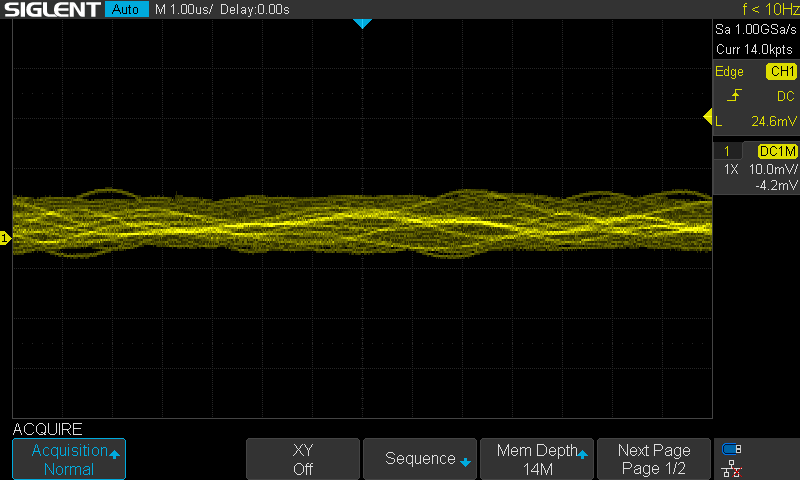3. Now, let’s enable averaging. For continuous (DC) outputs or repeated waveforms, averaging can be used to minimize random noise: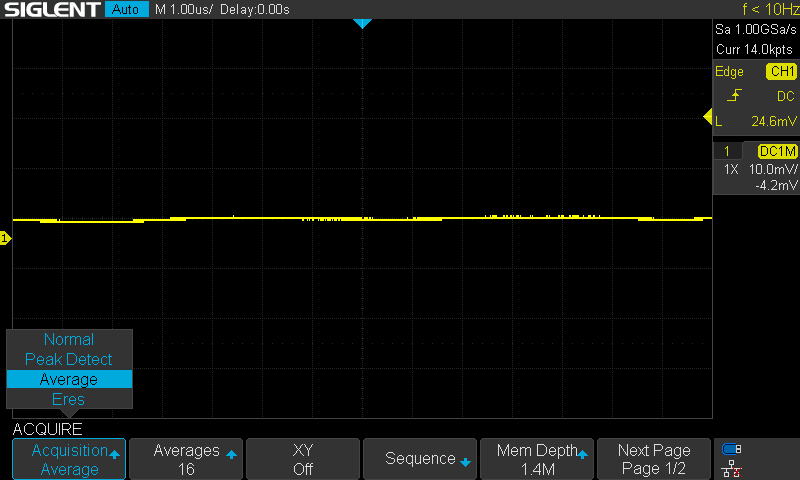4. We can also use the Zero function of the probe to minimize the DC offset of the measurement. Now, our open-circuit voltage average is near 0 V.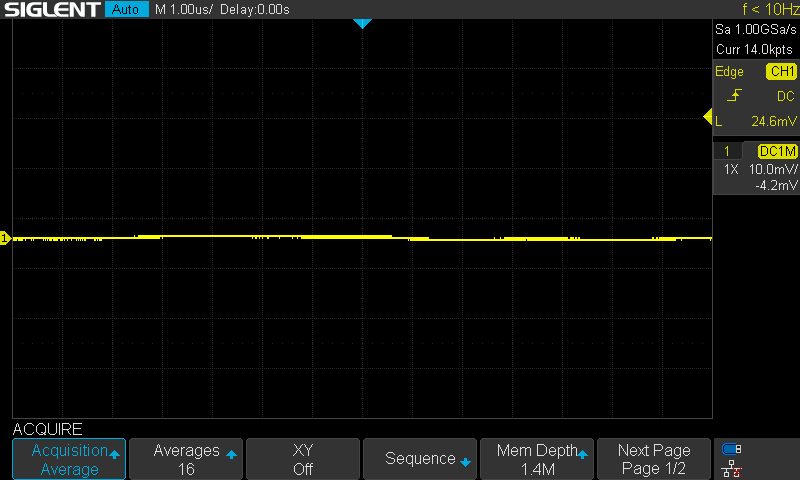5. Now, we can set the Units of Channel 1 to Amps (as we are measuring current) by pressing 1 to open the channel options menu. Set units to A and then select a custom scale factor so that the proper readings are displayed on the oscilloscope.

The probe is set to the 50 mV/A gain setting.

The scope always measures Volts.. so we would like to get this into Amps-per-Volt (A/V) so that we are converting the input signal (in Volts) to current (Amps)

The inverse of the probe gain is 1/(50 mV/A) = 0.02 A/mV * 1000 mV/V = 20 A/V

So, a 1 A current will provide a voltage at the scope of 50 mV/A * 1 A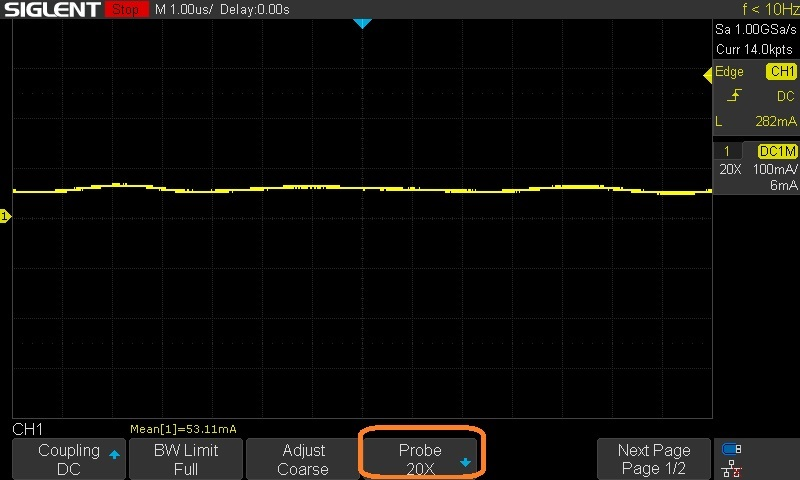6. Now, test the setup by sourcing a simple DC current with a power supply and a suitable wire to carry the current: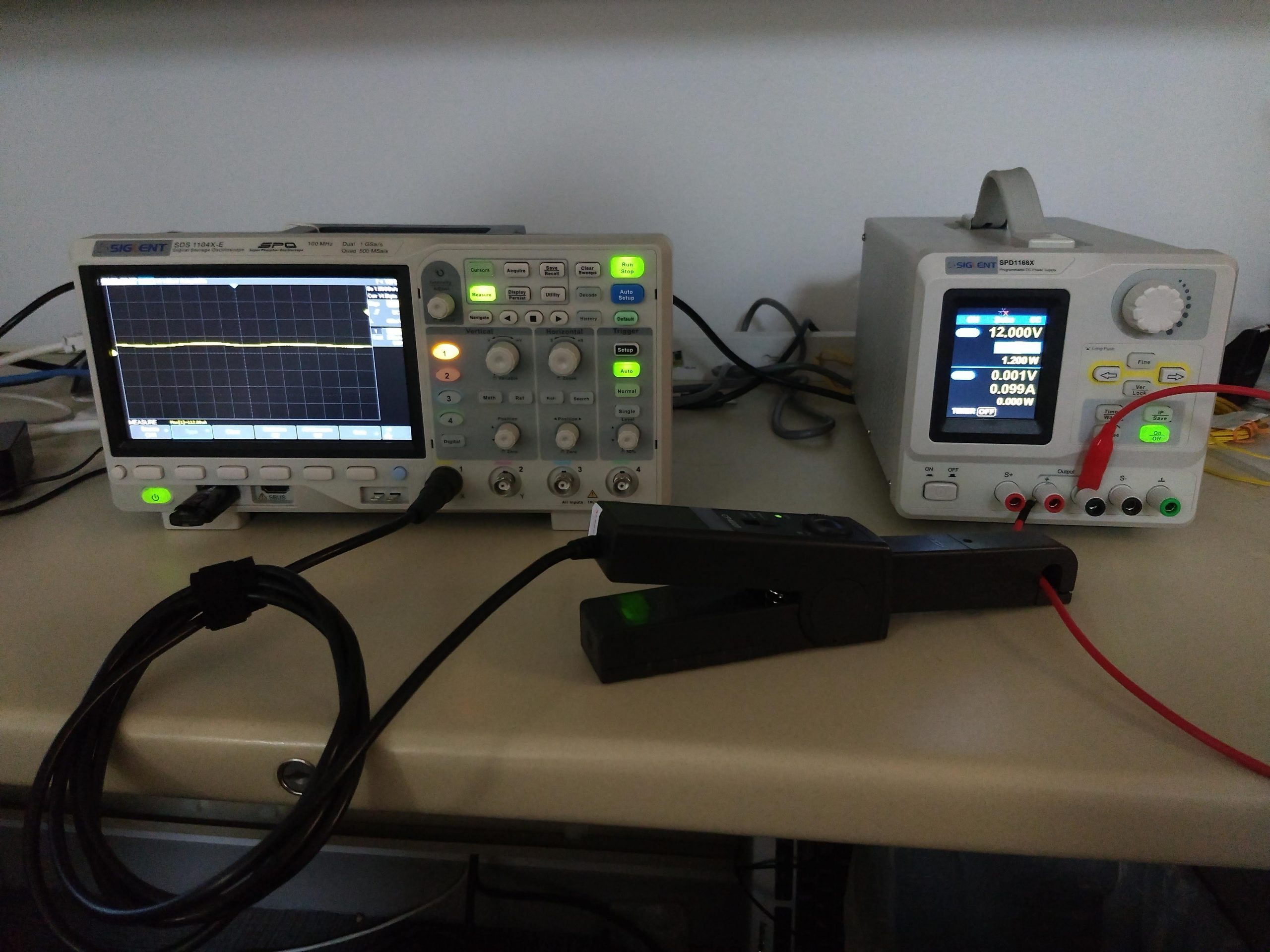I enabled a mean measurement which shows the value of the average signal input: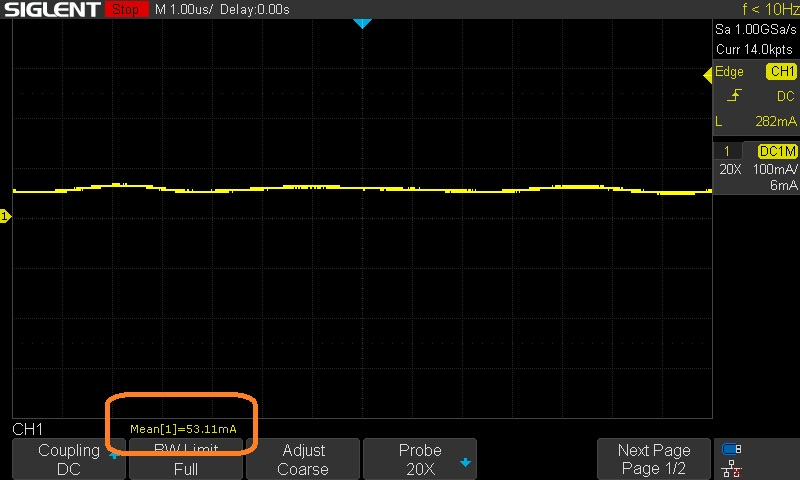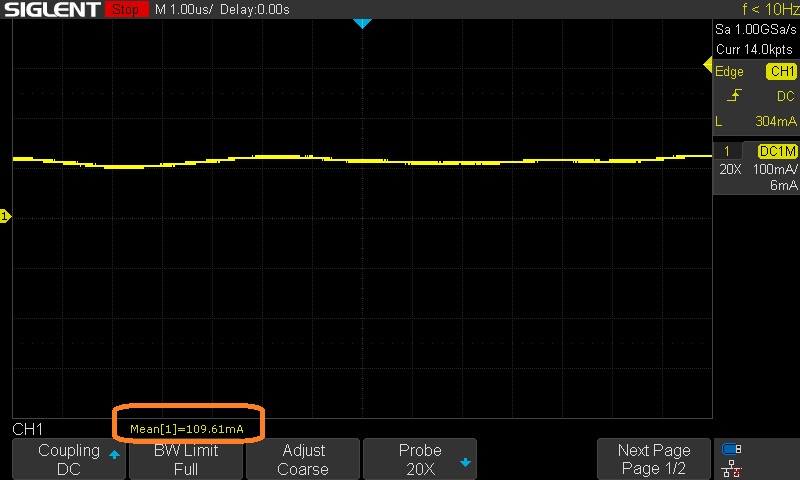The final estimate is that the CP4020 and XE scope have a low current measurement of approximately 50 mA.Welcome to SIGLENT North America.
Please select a website to continue

TW